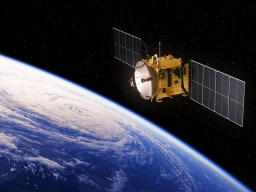# The satellite

The satellite orbiting the Earth at an altitude of 800 km has a speed of 7.46 km/s. For how long would it have to move from the start to the orbit to reach this speed if it evenly accelerated its motion in a straight line? What is the acceleration of satellite movement?

s
m/s2Did you find an error or inaccuracy? Feel free to write us. Thank you!Tips to related online calculators
Do you have a system of equations and looking for calculator system of linear equations?
Do you want to convert time units like minutes to seconds?